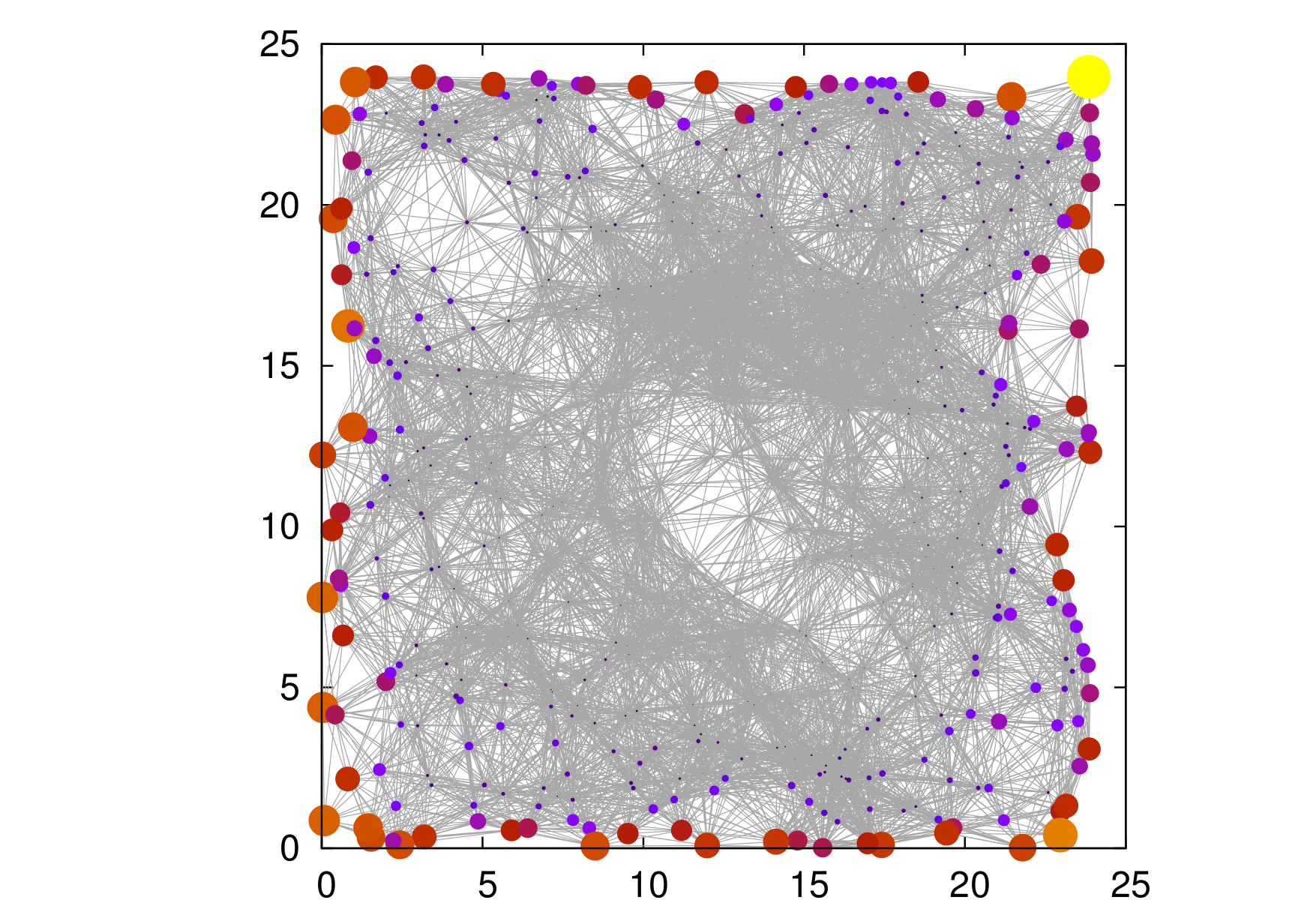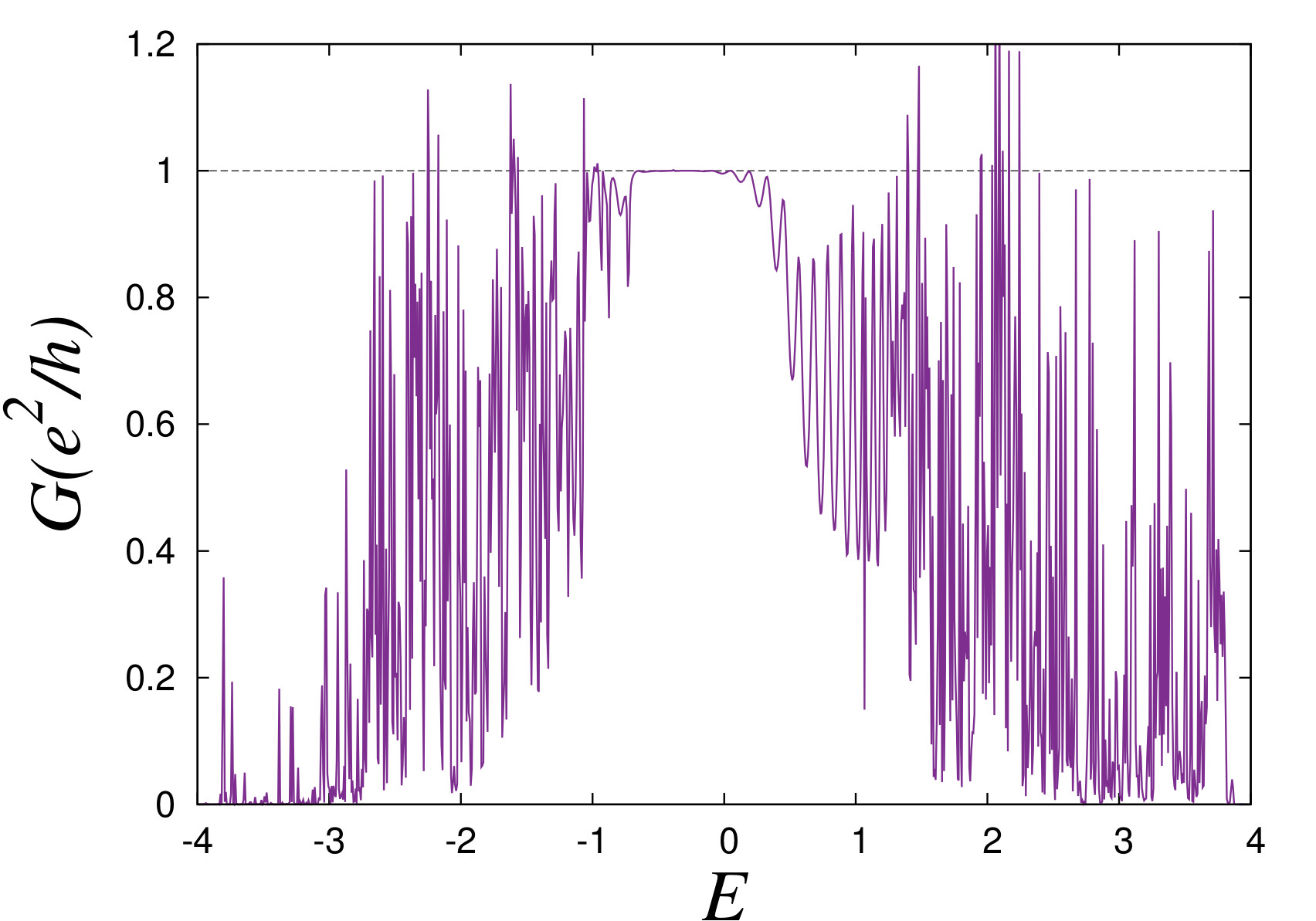# Study at IISc shows that glasses can be topological insulators

Usual materials come in two varieties. One, which will give you a shock if you connect it to the power socket — these are “metals”. Another, which will save you from the same shock — these are “insulators”. Aluminium, copper, iron are some common examples of the former kind. Rubber, wood belong to the latter kind. About a decade ago, theoretical physicists predicted that another type of material should exist. In these, if you apply some current, the current will be forced to move only on the surface. Inside, its still an insulator. Such materials are called topological insulators. The theoretical works predicting these materials were based on crystals — where atoms are arranged in a regular periodic fashion and scientists searched to find crystals with these properties. This theoretical work at IISc (see reference below) shows that this assumption is not necessary, topological insulators can exist even in an amorphous system, where atoms are arranged randomly!Numerical simulations show that a electronic wavefunction resides only on the edge of the simulated amorphous system in the topological phase.

Why is this important? Topological insulators has been long predicted and experimental labs all over the world have been competing to find such materials but with little real success (i.e. topological insulators that would find applications). The present study suggests that amorphous materials could host topological phases; vastly expanding possible candidate systems. The study also raises some fundamental questions for theory.Numerically calculated conductance from such a random lattice shows a value of e^2/h. e is the charge of an electron and h is the planck’s constant. Both are fundamental constants of nature. That the conductance shows this unique value is a hallmark of a topological phase.

https://arxiv.org/abs/1701.00374

website URL: